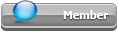## Featured Articles

Check out the latest featured articles.## File Library0# How Does Hysys Calculate Api Gravity?

hysys api gravity

|

### #1sam00

sam00

Brand New Member

•• Members
•• 1 posts

Posted 24 April 2019 - 09:18 AM

I have to generate API gravity values for the various stages of separation in my simulation.

I am calculating the API gravity based on the 'Liq. Mass Density (Std. Cond)' value for density.

However, Hysys can also also provide a value for the API gravity as part of the 'Petroleum Properties' property correlation. The API gravity that Hysys predicts is slightly different to what I calculate and appears (through back calculation) to be based on the 'Std. Ideal Liq. Mass Density' value.

Which is the correct method? Determine the API gravity from the 'Liq. Mass Density (Std. Cond)' or 'Std. Ideal Liq. Mass Density'?

I would have assumed that the value as determined through a non-ideal equation of state would be more representative of the 'true' API gravity of the oil.

Edited by sam00, 24 April 2019 - 09:22 AM.

### #2PaoloPemi

PaoloPemi

Gold Member

•• Members
•• 481 posts

Posted 25 April 2019 - 01:46 AM

the correlation which I use is 141.5 / Specific Gravity - 131.5
where Specific Gravity = crude oil density / 999
where liquid density is the density of crude oil at 60 F and 999 the density of water at 60 °F ,

normally I calculate oil density with a (extended, for liquid phase properties) EOS (for example PRX in Prode) but Aspen has some different models, anyway errors should be below 2 %

### Similar Topics